﻿ Calculation method > Xia-Bertoni

# XIA-BERTONI

Navegación:  Calculation method > Xia-Bertoni

# XIA-BERTONI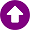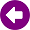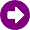DESCRIPTION

The Xia-Bertoni model [1-4] is an analytical method that allows estimating the basic propagation loss for urban and suburban scenarios. In comparison to similar models, as Walfish-Bertoni or COST 231, it can be used to predict the path loss for three different propagation scenarios with base-station antenna above, below, and near the average rooftop level. Hence, it is valid for both macro- and microcellular propagation environments.

The model identifies three propagation processes as the most important components which govern radio propagation in urban and suburban environments:

1) Free-space propagation.

2) Roof-street diffraction between the final diffraction building (FDB) and the mobile station.

3) Multi-screen diffraction due to the wave interaction with the buildings interposed between base station and the FDB.

The main contribution of this model is the analytic approach used to solve multiple forward diffraction past rows of buildings. The buildings rows are approximated as absorbing half-screens, so that the propagation over the buildings is treated as a multiple process forward diffraction past many half screens.

Fig. 1 shows a typical radio path and the geometrical parameters involved in path-loss prediction. It does not consider the orientation road angle respect to the direct radio path.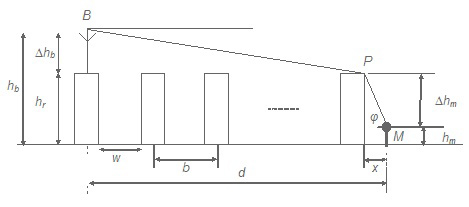Figure 1: Typical scenario for Xia-Bertoni model, for Δhb >> 0.

DEVELOPMENT

Considering the previously mentioned propagation processes, the total path loss, Lb, is expressed as the sum of three independent terms: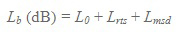(1)

where L0 is the free-space loss, Lrts is the diffraction loss from rooftop to street, and Lmsd is the reduction due to plane-wave multiple diffraction past rows of buildings.

Free space loss, L0

The free-space loss, L0, can be simply written as a function of frequency and transmitter-to-receiver distance. Following the expressions for three different cases:

i)  Base station antenna above rooftop level, hb >> hr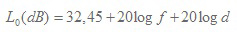(2)

where f is an MHz and d in km.

ii) Base station antenna near rooftop level, hb ≈ hr

An empirical factor of √2 (3 dB) which takes into account the contribution of local scattering from obstacles surrounding the base station that reinforces the signal in the forward direction. Hence, the equation (2) is rewritten as:(3)

iii) Base station antenna below rooftop level, hb< hr

The value of L0 is calculated by equation (3).

Roof-to-street loss, Lrst

Using the well established Geometrical Theory of Diffraction (GTD), Lrst is given by: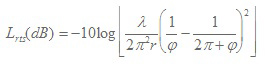(4)

where: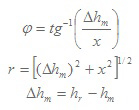r: distance from the diffraction point P to the mobile station M.

Δhm:  difference between the average rooftop level (hr) and the mobile station antenna (hm).

x: horizontal distance from the mobile station to the last diffraction edge. In general x=w/2, where w is the street width.

Developing equation (4) and expressing it as a function of the frequency f (MHz):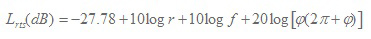(5)

Multiscreen diffraction loss, Lmsd

The multiscreen diffraction Lmsd is estimated by the following general expression: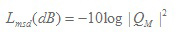(6)

The parameter QM is calculated by a multiple integration method including Boersma’s functions .

i) Base station antenna above rooftop level, hb >> hr

The value of QM is approximated by the expression: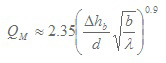(7)

where: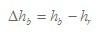Based on exhaustive measurement campaigns carried out by the model’s authors, the expression (7) was modified as follows: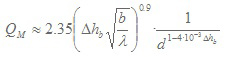(8)

By replacing (3.8) in (3.6), the expression below is obtained for multiscreen diffraction loss Lmsd: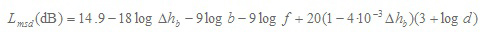(9)

where d is expressed in km, b in m and f and MHz.

ii) Base station antenna near rooftop level hb ≈ hr

This scenario corresponds to base station antennas placed 2-3 m above the average rooftop level. The parameter Δhb is approximated as Δhb ≈ 0 and the solution for QM proposed by Xia-Bertoni is the following: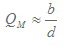(10)

and thus: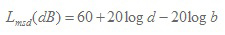(11)

where the distance d is expressed in km and b in m.

iii) Base station antenna below rooftop level hb< hr

Base-station antennas lower than the average rooftop level, are generally employed for microcellular applications, where the antenna is placed on the facade of the buildings, and thus Δhb< 0 (see Fig. 2).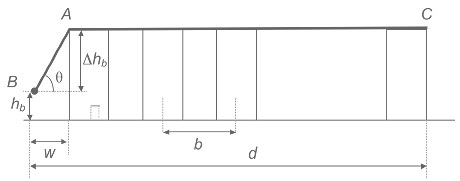Figure 2: Typical propagation scenario for Δhb < 0.

The process of multiple diffraction can be separated into two simpler wave diffraction processes. First (see BA path in Fig.2), the wave emitted is diffracted by the first row of buildings and it is treated by GTD as in the previous case of diffraction from rooftop-to-street. Second (see AC path in Fig.2) a multiple diffraction process past the remaining rows of buildings, approximated as the multiple diffraction of an array of absorbing half-screens. An exhaustive mathematical procedure  allows obtaining the term  Q2M expressed as follows: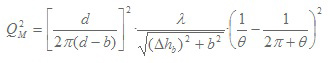(12)

Replacing the value of Q2M in (6), under the assumption of d-b ≈ d, the multiple screen diffraction loss, Lmsd, is expressed as: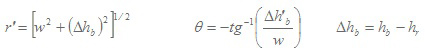(13)

where d is in km, f in MHz, r’ and b in m, and θ in radians, being:Basic propagation loss, Lb

Based on the previous study of the partial losses involved in the basic path loss estimation, below expressions summarize the method to calculate the total path loss, Lb, according to Xia-Bertoni model for the three different scenarios:

i) Base station antenna above rooftop level, hb >> hr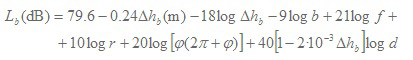(14)

REFERENCES

 H. H. Xia and H. L. Bertoni, "Diffraction of cylindrical and plane waves by an array of absorbing half-screens," in IEEE Transactions on Antennas and Propagation, vol. 40, no. 2, pp. 170-177, Feb 1992. doi: 10.1109/8.127401

 H. H. Xia, "A simplified analytical model for predicting path loss in urban and suburban environments," in IEEE Transactions on Vehicular Technology, vol. 46, no. 4, pp. 1040-1046, Nov 1997. doi: 10.1109/25.653077

 L. R. Maciel, H. L. Bertoni and H. N. Xia, "Unified approach to prediction of propagation over buildings for all ranges of base station antenna height," in IEEE Transactions on Vehicular Technology, vol. 42, no. 1, pp. 41-45, Feb 1993. doi: 10.1109/25.192385

 H. H. Xia, H. L. Bertoni, L. R. Maciel, A. Lindsay-Stewart and R. Rowe, "Microcellular propagation characteristics for personal communications in urban and suburban environments," in IEEE Transactions on Vehicular Technology, vol. 43, no. 3, pp. 743-752, Aug 1994. doi: 10.1109/25.312772